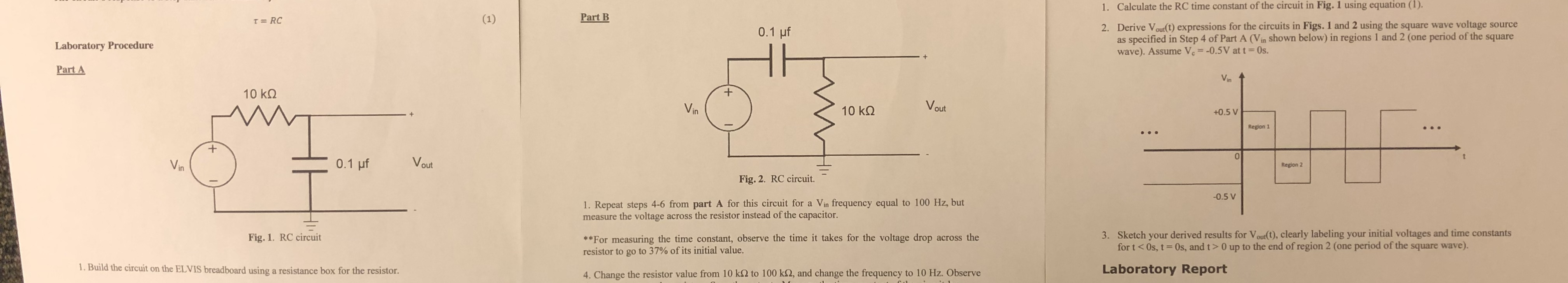# Calculate the RC time constant of the circuit in Fig. 1 using equation (1). 1. Part B Derive V oult) expressions for the circuits in Figs. 1 and 2 using the square wave voltage source as specified in Step 4 of Part A (Vin shown below) in regions 1 and 2 (one period of the square wave). Assume V.--0.5V at t 0s. 2. 0.1 uf Laboratory Procedure Part A 10 kΩ ka V Vin 10 kΩ +0.5 V Region 1 0 Region 2 Fig. 2. RC circuit. 0.5 V 1. Repeat steps 4-6 from part A for this circuit for a Vin frequency equal to 100 Hz, but measure the voltage across the resistor instead of the capacitor 3. Sketch your derived results for Vour(t), clearly labeling your initial voltages and time constants for t < 0s, t = 0s, and t > 0 up to the end ofregion 2 (one period of the square wave). *For measuring the time constant, observe the time it takes for the voltage drop across the resistor to go to 37% of its initial value. Fig. 1. RC circuit . Build the circuit on the ELVIS breadboard using a resistance box for the resistor Laboratory Report . Change the resistor value from 10 k92 to 100 k32, and change the frequency to 10 Hz. Observe

Question

Can someone help me with question 2 please?

Derive  Vout(t) expression for the circuit in figure 1 and figure 2 using the square wave voltage source as specific in Step 4 of part A (Vin shown below) in region 1 and region 2 (one period of the square wave). Assume Vc = -0.5 V at t=0shelp_outlineImage TranscriptioncloseCalculate the RC time constant of the circuit in Fig. 1 using equation (1). 1. Part B Derive V oult) expressions for the circuits in Figs. 1 and 2 using the square wave voltage source as specified in Step 4 of Part A (Vin shown below) in regions 1 and 2 (one period of the square wave). Assume V.--0.5V at t 0s. 2. 0.1 uf Laboratory Procedure Part A 10 kΩ ka V Vin 10 kΩ +0.5 V Region 1 0 Region 2 Fig. 2. RC circuit. 0.5 V 1. Repeat steps 4-6 from part A for this circuit for a Vin frequency equal to 100 Hz, but measure the voltage across the resistor instead of the capacitor 3. Sketch your derived results for Vour(t), clearly labeling your initial voltages and time constants for t < 0s, t = 0s, and t > 0 up to the end ofregion 2 (one period of the square wave). *For measuring the time constant, observe the time it takes for the voltage drop across the resistor to go to 37% of its initial value. Fig. 1. RC circuit . Build the circuit on the ELVIS breadboard using a resistance box for the resistor Laboratory Report . Change the resistor value from 10 k92 to 100 k32, and change the frequency to 10 Hz. Observe fullscreen

## Expert Answer

1 Rating

### Want to see this answer and more?

Experts are waiting 24/7 to provide step-by-step solutions in as fast as 30 minutes!*

*Response times vary by subject and question complexity. Median response time is 34 minutes and may be longer for new subjects.
Tagged in© 2021 bartleby. All Rights Reserved.